I don’t understand a question from your book. Question #2 on functions. Could you explain it in more detail?

The question: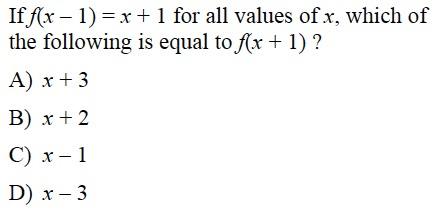I like to approach this by thinking about functions abstractly. A function is a process by which the thing in the parenthesis (AKA the argument) is transformed into something else using only mathematical operations. In this case, for all values of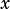, some mathematical operation or operations are done to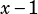to transform it into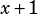.

What could those operations be? You can rule out multiplication or division—the leading coefficient of bothandis 1. Likewise, you can easily rule out exponents or roots. But what about addition/subtraction? Could something be added to or subtracted fromto transform it into? Sure! If you add 2 to, you get.

So let’s think about how that works in function notation. If we want to say that the function adds 2 to its input, we can write: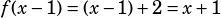That tells us whatwould be: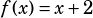And of course from there, we can see whatwould be: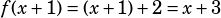Does that help?